# Fermat-Torricelli problem

(Redirected from Fermat–Torricelli problem)

Torricelli–Fermat problem

The (generalized) Fermat–Torricelli problem, incorrectly also called the Steiner–Weber problem, refers to finding the unique point,, minimizing the function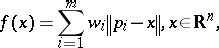(a1)

for a setofgiven non-collinear points with corresponding positive weights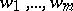, where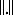denotes the Euclidean norm.

In 1638, R. Descartes invited P. de Fermat to investigate (for) curves of the form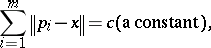(a2)

which led Fermat to ask in 1643 for the minimum point with respect to the unweighted subcase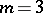of (a1) (i.e.,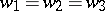), cf. [a14], p. 153. The first solution to this subcase was obtained by E. Torricelli (see [a29], [a30]) using the focal property of the ellipse, and further ruler-and-compass constructions were given by B. Cavalieri, V. Viviani, Th. Simpson, and H. Lebesgue; the unweighted case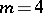was solved by G. Fagnano, cf. [a34], [a20], and [a2], Chap. II, for extensive historical discussions and corrections. With the help of Galois theory it was proved in [a6] and [a1] that for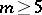points in general position the Fermat–Torricelli problem does not allow exact algorithms under computation models with arithmetic operations and extraction ofth roots. On the other hand,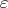-approximative solutions forcan be constructed in polynomial time, see [a4]. The weighted casewas completely solved by W. Launhardt [a21]; its relations to generalizations of the Napoleon theorem (also for higher dimensions) were investigated in [a24]. In 1846, E. Fasbender [a13] proved that the unweighted caseof minimizing (a1) is dual to the construction of the largest equilateral triangle circumscribed to the triangle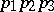, and H.W. Kuhn [a19] pointed out that this Vecten–Fasbender duality is historically the first example of dualizing a problem in the spirit of non-linear programming.

For, the level curves (a2) of the function (a1) (with equal weights) are called poly-ellipses or multifocal ellipses. They were first studied by E.W. von Tschirnhaus [a31], who extended the classical gardener's string construction fromin (a2) to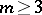. Many further properties and applications of these curves (and of their analogues in the weighted and higher-dimensional situation) are collected in [a25], [a12] and [a16].

Analytic approaches to the minimum pointof (a1) were presented by J. Bertrand, R. Sturm, L.L. Lindelöf, Kuhn, C. Witzgall, and many others; in modern terms they can be summarized by the following theorem (see, e.g., [a18]):

I) The minimum pointof (a1) exists and is unique.

II) If for each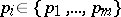,holds, then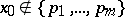and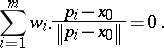III) If there is a pointsatisfying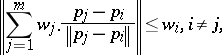then.

These characterizations ofhave a realistic appeal, since (for) there is even a mechanical device based on the so-called Varignon frame: A board is drilled withholes at the points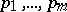;strings are tied together in a knot at one end, the loose ends are passed through theholes and are attached to physical weights below the board. The equilibrium position of the knot yields the solution. This was presented by G. Pick in [a32], Math. Appendix, but the early history of this approach is discussed in [a15].

The first algorithmic approach towas provided by E. Weiszfeld [a33], see also [a18]. It is gradient descent but not convergent at the foci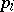(cf. also Gradient method). L.M. Ostresh [a27] proposed the first completely convergent iteration procedure, which can even be applied in Banach spaces, see [a11].

The Fermat–Torricelli problem has been one of the starting points of location science from operations research, in particular belonging to the field of continuous location theory, where it is usually called the-median problem or single facility location problem, cf. [a8], [a22], and from the historical point of view also [a21], [a32] (see also Weber problem).

Since local conditions for Steiner points in Steiner minimal trees (cf. also Steiner tree problem; Steiner point) are based on properties of Fermat–Torricelli points, a related passage in [a7], pp. 354–361, can be considered as starting point of investigations in this direction (although the respective question goes back even to C.F. Gauss, 1836: To connect four towns by a network of minimal total length). For historical corrections with respect to Steiner minimal trees (including the fact that even the name is not justified, analogous to the wrong phrase "Steiner–Weber problem" instead of "Fermat–Torricelli problem" ) see [a20] and [a2], Sect. 23.9; a modern treatment of Steiner minimal trees is [a5].

## Generalizations.

Generalizations of the problem to minimize (a1) were mainly studied in two directions.

Extensions to finite-dimensional normed spaces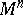(i.e., Minkowski spaces) or other non-Euclidean spaces: For example, denoting bythe (in Minkowski spaces not necessarily point-shaped) solution set, the property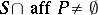holds for all finite point sets,, if and only ifis an inner product space [a9]. Various further properties of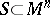were investigated in [a10], see also [a3]. Extensions to other non-Euclidean spaces are considered in [a11], [a26].

Modification of the given geometric configuration: For example, replacing the searched point in (a1) by a hyperplane, one gets the median hyperplane problem (also called linear fit problem, or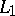regression problem), which can be formulated with respect to vertical and orthogonal distances. The importance of this problem (e.g., compared with the known least-squares regression) for robust statistics is based on the fact thatestimates are technically robust against arbitrary outliers, cf. [a28]. Also, such problems are studied in linear approximation theory and computational geometry (also in relation to the-set problem). Position criteria for median hyperplanes of weighted point sets and algorithmical approaches are presented in [a17] (for Euclidean-spaces) and in [a23] (for other Minkowski-spaces).